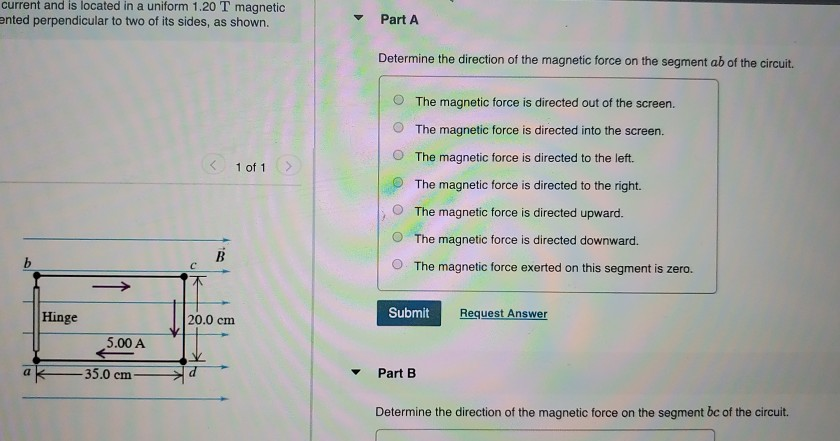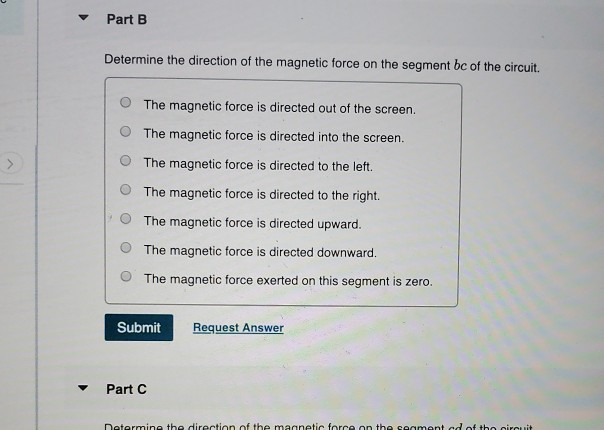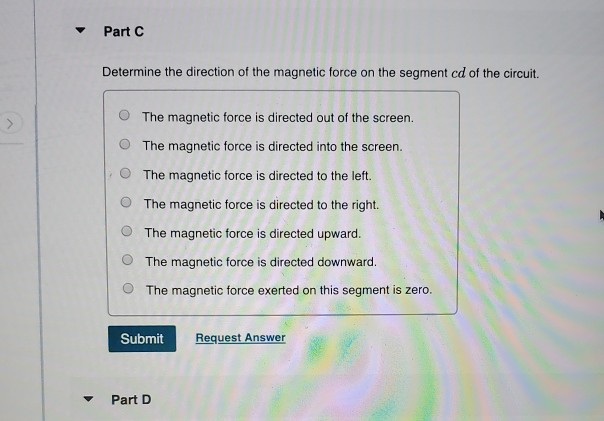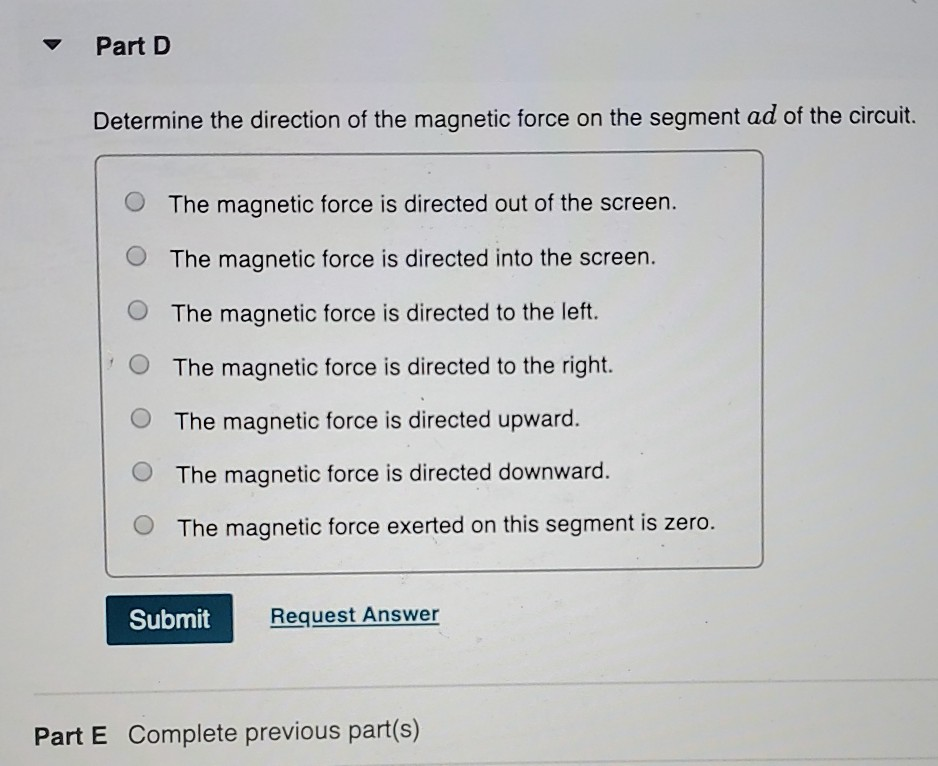# Question current and is located in a uniform 1.20 T magnetic ented perpendicular to two of its sides, as shown. Part A Determine the direction of the magnetic force on the segment ab of the circuit. The magnetic force is directed out of the screen. The magnetic force is directed into the screen. The magnetic force is directed to the left. 1 of 1 The magnetic force is directed to the right. The magnetic force is directed upward. O The magnetic force is directed downward. O The magnetic force exerted on this segment is zero. IT ||20.0 cm Submit Request Answer - Hinge 5.00 A 35.0 cm a Part B Determine the direction of the magnetic force on the segment bc of the circuit. Part B Determine the direction of the magnetic force on the segment bc of the circuit. O The magnetic force is directed out of the screen. 0 The magnetic force is directed into the screen. 0 The magnetic force is directed to the left. 0 The magnetic force is directed to the right. 0 The magnetic force is directed upward. The magnetic force is directed downward. O The magnetic force exerted on this segment is zero. Submit Request Answer Part C Determine the direction of the magnetic force on the carmant ed of the circuit Part C Determine the direction of the magnetic force on the segment cd of the circuit. The magnetic force is directed out of the screen. O The magnetic force is directed into the screen. The magnetic force is directed to the left. The magnetic force is directed to the right. The magnetic force is directed upward. The magnetic force is directed downward. The magnetic force exerted on this segment is zero. Submit Request Answer - Part D Part D Determine the direction of the magnetic force on the segment ad of the circuit. The magnetic force is directed out of the screen. O The magnetic force is directed into the screen. o O The magnetic force is directed to the left. O The magnetic force is directed to the right. O The magnetic force is directed upward. O The magnetic force is directed downward. O The magnetic force exerted on this segment is zero. Submit Request Answer Part E Complete previous part(s)Transcribed Image Text: current and is located in a uniform 1.20 T magnetic ented perpendicular to two of its sides, as shown. Part A Determine the direction of the magnetic force on the segment ab of the circuit. The magnetic force is directed out of the screen. The magnetic force is directed into the screen. The magnetic force is directed to the left. 1 of 1 The magnetic force is directed to the right. The magnetic force is directed upward. O The magnetic force is directed downward. O The magnetic force exerted on this segment is zero. IT ||20.0 cm Submit Request Answer - Hinge 5.00 A 35.0 cm a Part B Determine the direction of the magnetic force on the segment bc of the circuit. Part B Determine the direction of the magnetic force on the segment bc of the circuit. O The magnetic force is directed out of the screen. 0 The magnetic force is directed into the screen. 0 The magnetic force is directed to the left. 0 The magnetic force is directed to the right. 0 The magnetic force is directed upward. The magnetic force is directed downward. O The magnetic force exerted on this segment is zero. Submit Request Answer Part C Determine the direction of the magnetic force on the carmant ed of the circuit Part C Determine the direction of the magnetic force on the segment cd of the circuit. The magnetic force is directed out of the screen. O The magnetic force is directed into the screen. The magnetic force is directed to the left. The magnetic force is directed to the right. The magnetic force is directed upward. The magnetic force is directed downward. The magnetic force exerted on this segment is zero. Submit Request Answer - Part D Part D Determine the direction of the magnetic force on the segment ad of the circuit. The magnetic force is directed out of the screen. O The magnetic force is directed into the screen. o O The magnetic force is directed to the left. O The magnetic force is directed to the right. O The magnetic force is directed upward. O The magnetic force is directed downward. O The magnetic force exerted on this segment is zero. Submit Request Answer Part E Complete previous part(s)
More
Transcribed Image Text: current and is located in a uniform 1.20 T magnetic ented perpendicular to two of its sides, as shown. Part A Determine the direction of the magnetic force on the segment ab of the circuit. The magnetic force is directed out of the screen. The magnetic force is directed into the screen. The magnetic force is directed to the left. 1 of 1 The magnetic force is directed to the right. The magnetic force is directed upward. O The magnetic force is directed downward. O The magnetic force exerted on this segment is zero. IT ||20.0 cm Submit Request Answer - Hinge 5.00 A 35.0 cm a Part B Determine the direction of the magnetic force on the segment bc of the circuit. Part B Determine the direction of the magnetic force on the segment bc of the circuit. O The magnetic force is directed out of the screen. 0 The magnetic force is directed into the screen. 0 The magnetic force is directed to the left. 0 The magnetic force is directed to the right. 0 The magnetic force is directed upward. The magnetic force is directed downward. O The magnetic force exerted on this segment is zero. Submit Request Answer Part C Determine the direction of the magnetic force on the carmant ed of the circuit Part C Determine the direction of the magnetic force on the segment cd of the circuit. The magnetic force is directed out of the screen. O The magnetic force is directed into the screen. The magnetic force is directed to the left. The magnetic force is directed to the right. The magnetic force is directed upward. The magnetic force is directed downward. The magnetic force exerted on this segment is zero. Submit Request Answer - Part D Part D Determine the direction of the magnetic force on the segment ad of the circuit. The magnetic force is directed out of the screen. O The magnetic force is directed into the screen. o O The magnetic force is directed to the left. O The magnetic force is directed to the right. O The magnetic force is directed upward. O The magnetic force is directed downward. O The magnetic force exerted on this segment is zero. Submit Request Answer Part E Complete previous part(s)